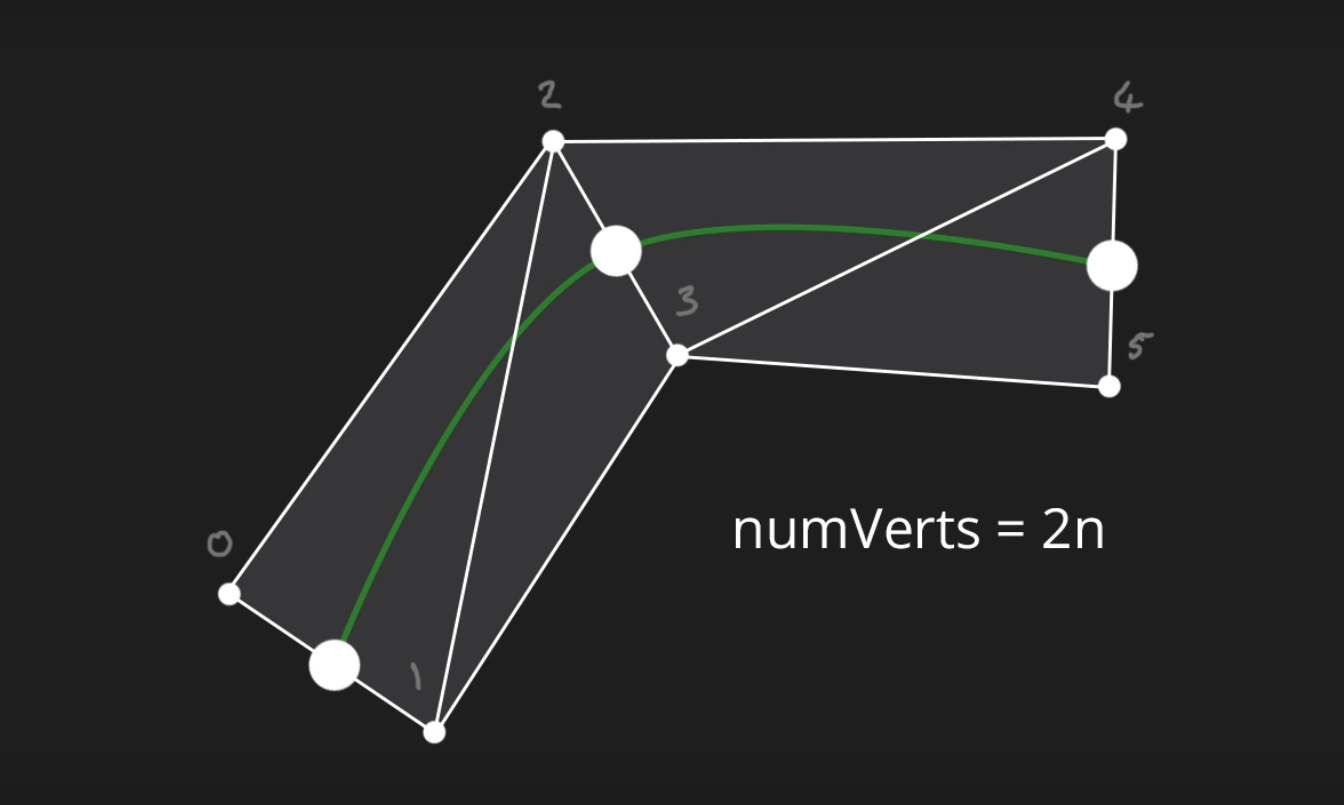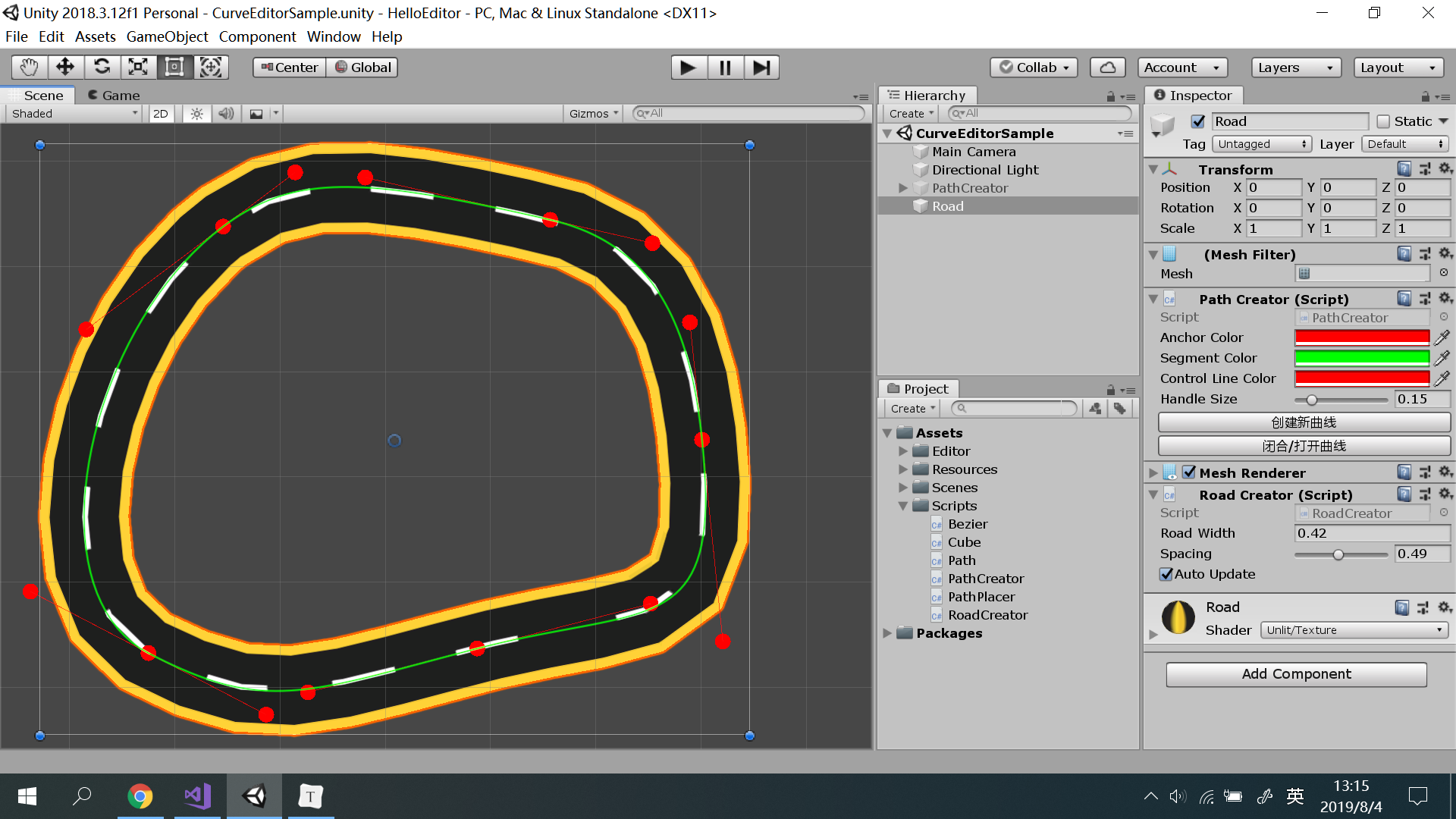Unity 2018.3.12f1Unknown LicenseUpdated 167 days agoCreated on July 25th, 2019
Go to source

# Hello Editor

Unity Editor

## Custom Inspector

`Cube` 脚本自定义 `Inspector`，可随机改变方块颜色与重置。(`Scenes/CubeEditorSample`)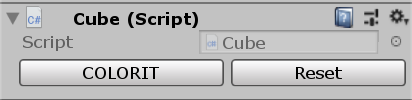## 曲线编辑器

### 已实现的功能

• 创建曲线
• Shift + 左键 添加节点
• 右键 删除节点
• 移动节点，锚点跟随
• 调整锚点，对应锚点方向跟随
• 按住 Ctrl 自由调整单个锚点
• 曲线闭合，首尾相接
• 计算曲线的平均分割点
• `Mesh` 的创建（`RoadCreator`

### 部分具体实现

#### 1. Path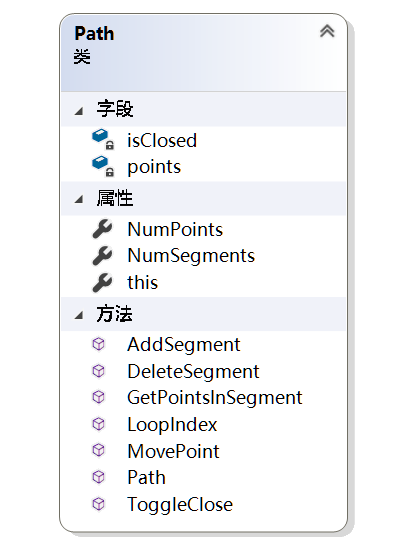#### 2. 创建曲线

``````public Path(Vector2 center) {
points = new List<Vector2>() {
center + Vector2.left,
center + (Vector2.left + Vector2.up) * 0.5f,
center + (Vector2.right + Vector2.down) * 0.5f,
center + Vector2.right
};
}
``````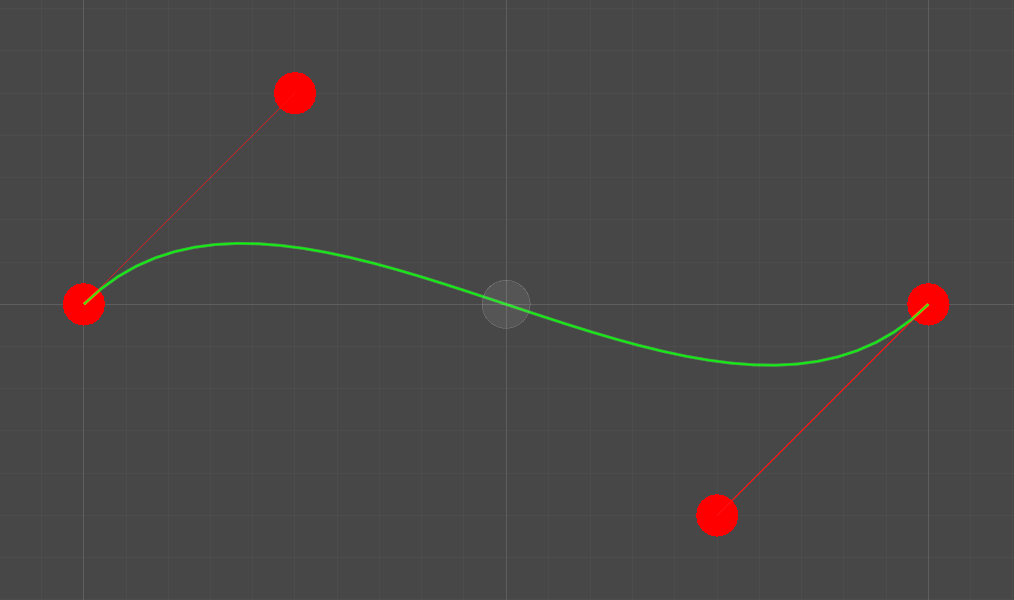#### 3. 添加节点

``````public void AddSegment(Vector2 seg) {
points.Add(points[points.Count - 1] * 2 - points[points.Count - 2]);
points.Add((points[points.Count - 1] + seg) / 2);
}
``````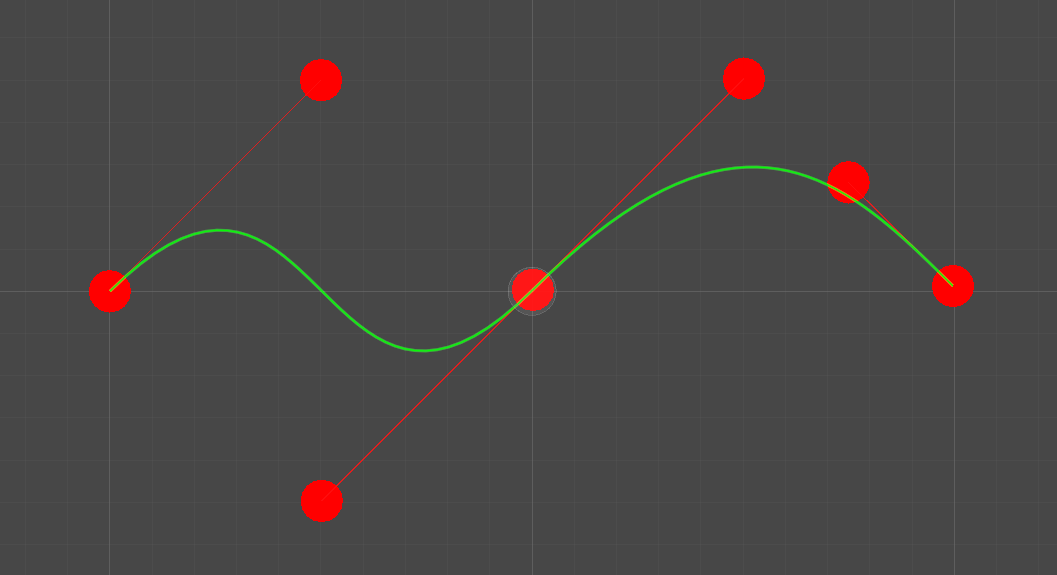#### 4. 删除节点

• 普通情况（删除中间某个节点）：只需删除节点左右两个点和节点本身，使用 `points.RemoveRange(index - 1, 3)` 即可。
• 删除节点：如果曲线未闭合则只需删除 `points` 的前三个点，即上图中的 `0、1、2`。如果曲线闭合则在删除这三个点之前将最后一个点的值改为 `points` 即新的首节点的第一个锚点。
• 删除节点：如果未闭合则需删除 `points` 的最后三个点，即上图中的 `4、5、6` ，使新的尾节点只有一个锚点。如果曲线闭合，则不需删除新的尾节点的第二锚点，而需要删除最后一个点（首节点的一个锚点）。
``````public void DeleteSegment(int index) {
if (NumSegments <= 2 && (isClosed || NumSegments <= 1))
return;

if (index == 0) {
if (isClosed)
points[points.Count - 1] = points;
points.RemoveRange(0, 3);
}
else if (index == points.Count - 1 && !isClosed) {
points.RemoveRange(index - 2, 3);
}
else {
points.RemoveRange(index - 1, 3);
}
}
``````

#### 5. 移动节点

• 单纯移动点的部分只需将坐标赋值给对应点即可。
• 移动节点时还要将对应的两个锚点同时移动，使其保持相对位置。
• 移动锚点时如果没有按下 Ctrl 则要将所相对的锚点同时移动，保持方向相对，但对面锚点到节点的距离不变。
``````public void MovePoint(int i, Vector2 pos) {
Vector2 offset = pos - points[i];
points[i] = pos;

switch (i % 3) {
case 0:
if (i - 1 >= 0 || isClosed)
points[LoopIndex(i - 1)] += offset;
if (i + 1 < NumPoints || isClosed)
points[LoopIndex(i + 1)] += offset;
break;
case 1:
if ((i - 2 >= 0 || isClosed) && !Event.current.control) {
float dst = (points[LoopIndex(i - 1)] - points[LoopIndex(i - 2)]).magnitude;
Vector2 dir = (points[LoopIndex(i - 1)] - pos).normalized;
points[LoopIndex(i - 2)] = points[LoopIndex(i - 1)] + dir * dst;
}
break;
case 2:
if ((i + 2 < NumPoints || isClosed) && !Event.current.control) {
float dst = (points[LoopIndex(i + 1)] - points[LoopIndex(i + 2)]).magnitude;
Vector2 dir = (points[LoopIndex(i + 1)] - pos).normalized;
points[LoopIndex(i + 2)] = points[LoopIndex(i + 1)] + dir * dst;
}
break;
default:
break;
}
}
``````

#### 6. 闭合曲线

``````public void ToggleClose() {
isClosed = !isClosed;

if (isClosed) {
points.Add(points[points.Count - 1] * 2 - points[points.Count - 2]);
}
else {
points.RemoveRange(points.Count - 2, 2);
}
}
``````

#### 7. 曲线和锚点的绘制

• 锚点的绘制用到了 `Handles` 类中的 `FreeMoveHandle()` 方法来绘制了一个圆柱形手柄，手柄移动后的位置返回给 `pos` ，通过这个返回的 `pos` 来移动点。在移动之前用 `Undo` 类记录了当前状态，可以撤销这一步的移动。
• 曲线的绘制用到了 `Path` 类中的 `GetPointsInSegment(int)` 方法来获取某一段曲线的个控制点，`0、1` 两个点连线，`2、3` 两个点连线，然后利用这四个点绘制一条从 `0``3` 的贝塞尔曲线。
``````private void Draw() {
// 绘制锚点
Handles.color = Color.red;
for(int i = 0; i < path.NumPoints; i++) {
Vector2 pos = Handles.FreeMoveHandle(path[i], Quaternion.identity, 0.1f, Vector3.zero, Handles.CylinderHandleCap);
if (pos != path[i]) {
Undo.RecordObject(creator, "移动锚点");
path.MovePoint(i, pos);
}
}

// 绘制曲线
for(int i = 0; i < path.NumSegments; i++) {
Vector2[] points = path.GetPointsInSegment(i);
Handles.DrawLine(points, points);
Handles.DrawLine(points, points);
Handles.DrawBezier(points, points, points, points, Color.green, null, 4f);
}
}
``````#### 9. Inspector GUI

`PathCreator``Inspector` 上添加了两个按钮：创建新曲线闭合/打开曲线 ，只需要将继承了 `Editor``PathEditor` 覆写 `OnInsepectorGUI()` 方法，在基类方法的基础上添加这两个按钮。点击按钮后通过 `SceneView` 类的 `RepaintAll()` 方法重新绘制。

``````public override void OnInspectorGUI() {
base.OnInspectorGUI();

if (GUILayout.Button("创建新曲线")) {
creator.CreatePath();
path = creator.path;
SceneView.RepaintAll();
}

if (GUILayout.Button("闭合/打开曲线")) {
path.ToggleClose();
SceneView.RepaintAll();
}
}
``````

#### 10. 用 Mesh 实现 RoadCreator

• 中间节点的红箭头方向是该节点到下一节点方向上一节点到该节点方向的向量和。
• 两端节点的红箭头方向则是其中仅有的一个方向的表示。
``````for (int i = 0; i < points.Length; i++) {
Vector2 forward = Vector2.zero;

if (i > 0)
forward += points[i] - points[i - 1];
if (i < points.Length - 1)
forward += points[i + 1] - points[i];

forward.Normalize();
}
``````

``````Vector2 left = new Vector2(-forward.y, forward.x);
``````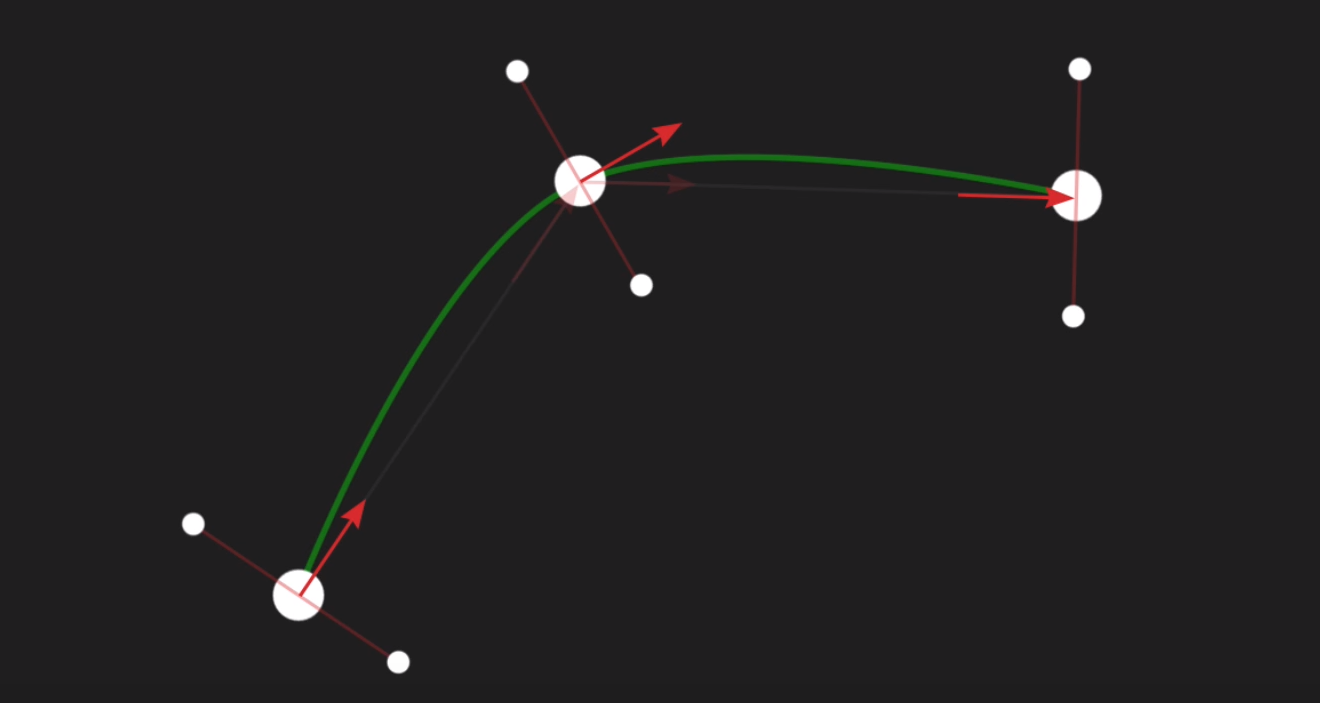`Mesh` 还需设置这些三角形，`mesh.triangles = tris`，即每个三角形对应的三个顶点坐标的集合。下图四个三角形中第一个三角形需从 `0-2-1` 的顺序，第二个三角形从 `1-2-3` 的顺序，顺序不对三角形会正反颠倒。

``````float percent = i / (float)(points.Length - 1);
percent = 1 - Mathf.Abs(percent * 2 - 1);	// 1-|2x-1| 使 x 从原来的 0->1 变成了 0->1->0